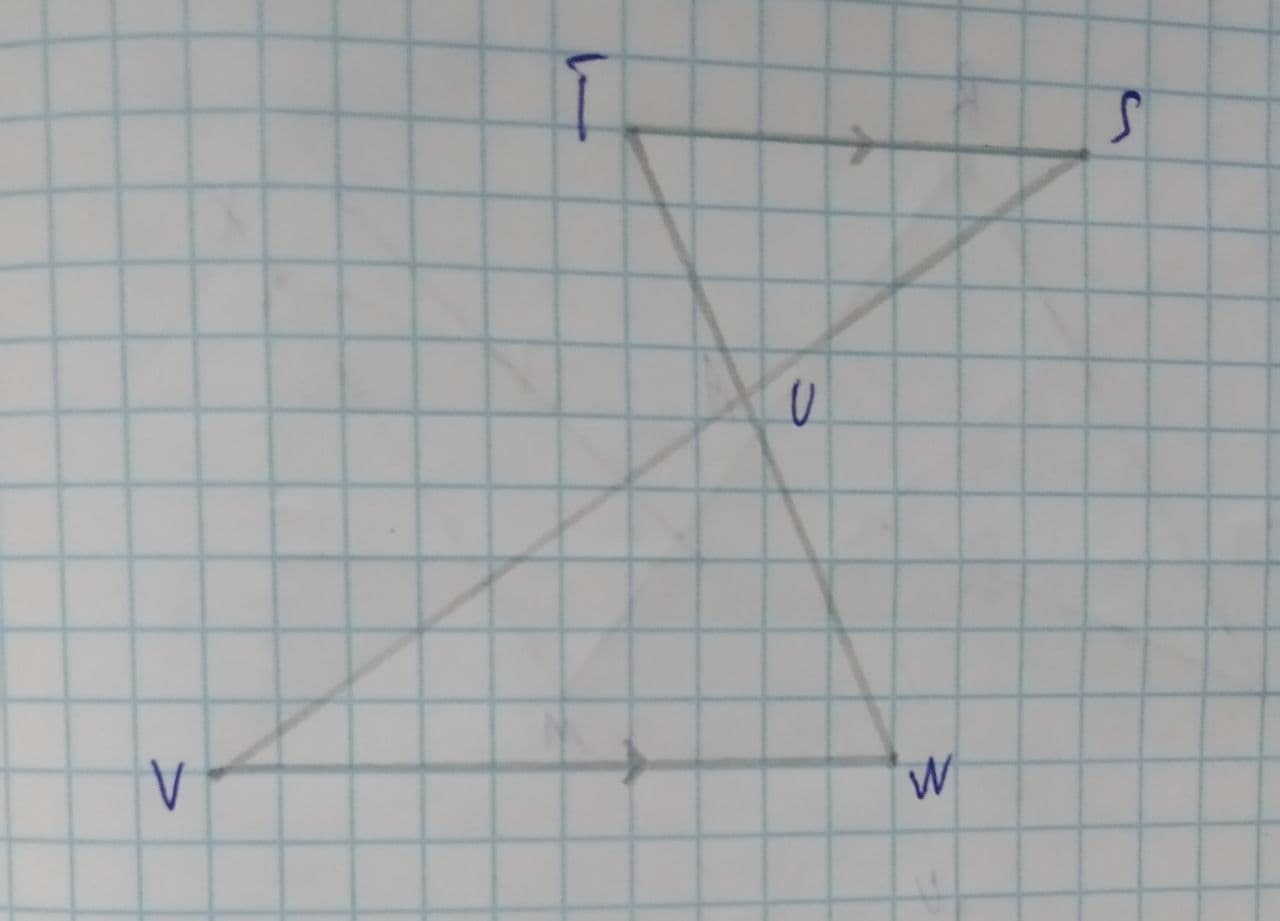# Determine whether the two triangles are similar \triangle TSU\sim \triangleClifland 2021-08-11 Answered
Determine whether the two triangles are similar. If they are, complete the similarity statement.
Select all that apply.
$$\displaystyle\triangle{T}{S}{U}\sim\triangle$$ ?The triangles are not similar.
$$\displaystyle\triangle{T}{S}{U}\sim\triangle{W}{V}{U}$$
$$\displaystyle\triangle{T}{S}{U}\sim\triangle{V}{W}{U}$$
By SAS similarity
By SSS similarity
By AA similarity

• Questions are typically answered in as fast as 30 minutes

### Plainmath recommends

• Get a detailed answer even on the hardest topics.
• Ask an expert for a step-by-step guidance to learn to do it yourself.Szeteib

Observe that
$$\displaystyle{T}{S} \parallel {V}{W}$$ (parallel)
In $$\displaystyle\triangle{T}{S}{U}\ {\quad\text{and}\quad}\ \triangle{W}{V}{U}$$
$$\displaystyle\angle{S}{T}{U}=\angle{V}{W}{U}$$ (Interior Alternate Angle)
Also $$\displaystyle{T}{S}{U}=\angle{U}{V}{W}$$ (Interior Alternate Angle)
So, $$\displaystyle\triangle{T}{S}{U}\sim\triangle{W}{V}{U}$$ by AA Similarity
Also $$\displaystyle\triangle{W}{V}{U}$$ in same $$\displaystyle\triangle{V}{W}{U}$$
Hence Correct options are
$$\displaystyle\triangle{T}{S}{U}\sim\triangle{W}{V}{U}$$
$$\displaystyle\triangle{T}{S}{U}\sim\triangle{V}{W}{U}$$
By AA similarity